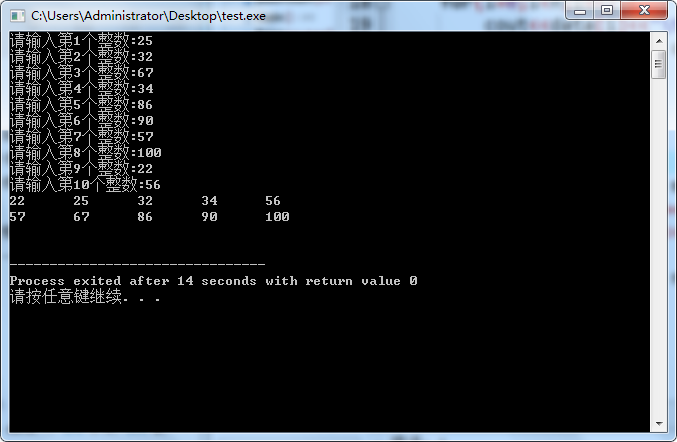• 【千律】C++基础：编写实现数组升序排列的函数
2022-03-17 15:06:39
#include <iostream>
using namespace std;

void MySort(int IntegerArray[], int length)
{
// 初始化中间变量
int InterVariables = 0;

for (int i = 0; i < length; i++)
{

// 对第i个数进行i次比较，依次前移
for (int j = i; j > 0; j--)
{

// 对前后两个数字进行比较，将数字较小的前移
if (IntegerArray[j] <= IntegerArray[j - 1])
{
InterVariables = IntegerArray[j];
IntegerArray[j] = IntegerArray[j - 1];
IntegerArray[j - 1] = InterVariables;
}

}
}
}
int main()
{

// 初始化数组
int IntegerArray = { 5, 8, 9, 0, 2, 1, 4, 7, 6, 3, 21};

// 得到数组长度
int length = sizeof(IntegerArray) / sizeof(IntegerArray);

// 数组升序排列
MySort(IntegerArray, length);

// 循环输出排序后的数组
for (int i = 0; i < length; i++)
{
cout << IntegerArray[i] << " ";
}
cout << endl;

return 0;
}

更多相关内容
• ## C++ | 数组升序插入

千次阅读 2019-10-07 17:54:45
编写一个插入排序函数InsertSort，三个参数：第一参数是含有n个元素的数组，这n个元素已升序排序；第二个参数是当前数组的元素个数；第三个参数是要插入的整数。 该函数功能实现一个整数插入到数组中，然后进行升序...

### 实验内容

实现插入排序方法，输入10个整数，按升序排序输出。编写一个插入排序函数InsertSort，三个参数：第一参数是含有n个元素的数组，这n个元素已升序排序；第二个参数是当前数组的元素个数；第三个参数是要插入的整数。
该函数功能实现一个整数插入到数组中，然后进行升序排序。
Print函数输出数组，一行5个元素

代码段

#include <iostream>
using namespace std;
void InsertSort(int data[], int & n, int a)
{
int i;
for(i=0; i<n; i++)
if(a<=data[i]) break;
if(i==n) data[n] = a;
else {
for(int j=n; j>i; j--)
data[j] = data[j-1];
data[i] = a;
}
n++;
}
void Print(int data[], int n) {
int i;
for(i=0;i<n;i++) {
cout<<data[i]<<"\t";
if((i+1)%5 == 0) cout<<endl;
}
cout<<endl;
}
int main()
{
int data, n = 0, m;
for(int i=0; i<10; i++) {
cout<<"请输入第"<<i+1<<"个整数:";
cin>>m;
InsertSort(data, n, m);
}
Print(data, n);
return 0;
}


### 实验结果展开全文• 代码如下： #include #include #include int main() { int SIZE=10,i,j; double a[SIZE],t;... printf("升序排列结果为："); for(i=0;iprintf("%.1lf ",a[i]); return 0; } 怎么回事，实在搞不懂了
• 数组升序排列后，插入一个数，仍然保持升序排列。 先进性逆序排序 java public static void main(String[] args) { // TODO Auto-generated method stub Scanner input = new Scanner(System.in); int[] ...

将数组升序排列后，插入一个数，仍然保持升序排列。

先进性逆序排序
java
public static void main(String[] args) {
Scanner input = new Scanner(System.in);
int[] scores = new int[]{99,85,82,63,60};
int i,j;

	//定义一个临时变量，将数组中第一个数赋给这个临时变量
int min =scores;

//利用循环，通过冒泡排序将大的数不断置换到数组后面，小的数置换到数组前面
for(i=0; i<scores.length; i++){
for(j=i+1; j<scores.length; j++){
if(scores[i]>scores[j]){
min = scores[j];
scores[j] = scores[i];
scores[i] = min;
}
}
}

//定义一个新数组，通过循环把老的数组里的数复制到新数组里
int[] newScores = new int;
for(i=0; i<scores.length; i++){
newScores[i] = scores[i];
}

//定义一个布尔值，作为标记
boolean a = false;

//数组下标
int index = 0;
System.out.println("请输入一个正整数：");
int num = input.nextInt();

//利用循环将插入的数与数组中的数进行比较
for(i=0; i<newScores.length-1; i++){
//当遇到数组中的数比这个数大时，记下此时数组中的数的下标，并且break.跳出循环
if(num<newScores[i]){
index = i;
break;
}
//当这个数比数组中所有的数都大时，将这个点标记为true,并将数组中最后一个下标索引赋予index
while(num>=newScores[newScores.length-2]){
a = true;
index = newScores.length-1;
break;

}
}
//当a为false时，用循环将插入位置的数及之后的数逐个向后移动一位(必须从最后一个数开始移动)
if(a==false){
for(i=newScores.length-1; i>index; i--)
{
newScores[i] = newScores[i-1];
}
数移动完之后再将这个数插入到已标记好的位置
newScores[index] = num;
//当a为true时，只需把数插入到数组的最后一位
}else{
newScores[index] = num;
}
//循环输出
for(i=0; i<newScores.length; i++){
System.out.print(newScores[i]);
}


}




展开全文• 方法1：冒泡排序 冒泡排序的原理是：从左到右，将相邻元素进行比较，每比较一轮，就会找到序列中最大的... 方法2：用sort方法排序 sort:是对容器或普通数组中 [first, last) 范围内的元素进行排序，默认进行升序排序。

### 方法1：冒泡排序

冒泡排序的原理是：从左到右，将相邻元素进行比较，每比较一轮，就会找到序列中最大的一个或最小的一个。

<script>
var arr = [1, 9, 4, 0, 6, 0, 11, 3, 2];
// 1.冒泡排序
function mySort(arr) {
// 外层循环控制比多少轮
for (var i = 0; i <= arr.length - 1; i++) {
// 内层循环控制一轮比较多少次
for (var j = 0; j <= arr.length - 1 - i; j++) {
if (arr[j] > arr[j + 1]) {
var temp = arr[j];
arr[j] = arr[j + 1];
arr[j + 1] = temp;
}
}
}
console.log(arr);
}
</script>

### 方法2：用sort方法排序

sort:是对容器或普通数组中 [first, last) 范围内的元素进行排序，默认进行升序排序。

<script>
var arr = [1, 9, 4, 0, 6, 11, 3, 2];
let newArr = arr.sort(function (a, b) {
return b - a
})
console.log(newArr);
</script>

展开全文javascript 排序算法
• 给定一个整数数组 nums，将该数组升序排列。 示例 1： 输入：[5,2,3,1] 输出：[1,2,3,5] 示例 2： 输入：[5,1,1,2,0,0] 输出：[0,0,1,1,2,5] 提示： 1 <= A.length <= 10000 -50000 <= A[i] <= 50000 ...
• 我在冒泡排序的函数里试着没有传递数组长度，而是在这个函数里用sizeof直接求出长度，如第二行所示，为什么结果不正确 如下图所示，没有得到正确的排序？c语言
• 1.按照频率将数组升序排列 //给你一个整数数组 nums ，请你将数组按照每个值的频率 升序 排序。如果有多个值的频率相同，请你按照数值本身将它们 降序 排序。 // // 请你返回排序后的数组。 // // // // 示例 ...
• 关于这个问题我给出了两个不同的方法，一是将二维数组看成一维数组，二是运用指针的思想来简化算法。 其实这个问题一个是可能不明白如何将二维数组看成一维数组（for example me...)再一个就是指针的问题了 哈哈哈 ...排序算法 开发语言
• #include #include #include int *sortIndex(int b[], int n) {  int *m;  int temp;...//此时输出的数组已经为排好序后的数组  printf("\n");    system("pause");  return ; }
• 今天在做下面这道题的时候糊涂了，把二维数组的排序做错了。 题目 假设有打乱顺序的一群人站成一个队列，数组 people 表示队列中一些人的属性（不一定按顺序）。每个 people[i] = [hi, ki] 表示第 i 个人的身高为 ...贪心算法 排序 lamda表达式
• 封装一个函数，利用冒泡排序，使数组升序排列 #include<iostream> using namespace std; void Sort(int* arr, int len) { for (int i = 0; i < 6 - 1; i++) { for (int j = 0; j < 6 - i - 1; j++)...
• 数组的元素按从小到大进行排序。 Format Input 有两行 第一行有一个整数n( 5 <= n <= 10 ) 第二行有n个整数 Output 输出更新后的数组 Samples 【输入样例】 输入数据 1 8 1 2 3 6 8 7 4 5 Copy...算法
• 案例：将数组{4，2，8，0，5，7，1，3，9}进行升序排列 1、比较相邻的元素。如果第一个比第二个大，就交换他们两个。 2、对每一对相邻元素做同样的工作，执行完毕后，找到第一个最大值。 3、重复以上的步骤，每次的...
• #include using namespace std; int main() { int s,x,i; cin>>x; for( i=0;i;i++) cin>>s[i]; for( i=0;i;i++) { if(s[i]>x) break; } for(int j=9;j>=i;j--) { s[j+1]=s[j]; } s[i]=x; for( i=0;... }
• 本文章简单介绍了sort的使用，包括升序排序、降序排序、对自定义类型（如结构体）的排序排序
• 一、升序C++ sort 函数十分方便，可以对内置类型也可对自定义类型进行快速排序，内置类型的使用比较简单，下面主要讨论自定义类型的排序，一般有如下几种使用方法：1.1 重载比较操作符比如，我们现有一批学生，要...
• #include<stdio.h> void sort(int x[],int n); int main() { int arr = { 11,3,7,23,15,79,66,58,69,45 },i; sort(arr, 10); printf("排序结果如下：\n"); for (i = 0; i <... ...
• 输入整型数组和排序标识，对其元素按照升序或降序进行排序（一组测试用例可能会有多组数据） 接口说明 原型： voidsortIntegerArray(Integer[]pIntegerArray,intiSortFlag); 输入参数： Integer[]pIntegerArray...
• #include一般用在C、C++等语系的编译环境（就是用在编程软件的编程代码里）中，直白的说，它就是告诉你，在这个地方，你要插入一堆代码，这堆代码在另一个文件里。 例如：#include这就是说，在代码的这个地方，要...
• 问题描述： 【项目2 - 删除数组元素并进行排序】 del函数的作用是删除数组a中的...然后进行升序排序，函数的原型为： int del (int a,int n,int x) 程序代码 #include #include #define N 50 /* *csdn学院--20
• bool FeatureCmp(Keypoint&amp; f1, Keypoint&amp; f2) {  return f1.scale &...//创建一维数组  size_t m=3; //假定数组长度为m double *matched; matched = new double[m]; //动态分配空间 //一...
• c语言给数组插入若干个数并令插入后的数列升序输出c#
• 题目描述：给你一个整数数组 nums，请你将该数组升序排列。 基本思想：通过一趟排序将待排序记录分割成独立的两部分，其中一部分记录的关键字均比另一部分记录的关键字小，则可分别对这两部分记录继续进行排序，以...快速排序 排序算法 数据结构
• 完整代码： ...实现一维数组升序排序； //函数定义： void maopao(int * p, int len)//也可写成int p[ ]；引入数组的首地址p=arr； { cout << "冒泡升序前的数组为：" << endl; for (i...
• ## C++将一维数组从小到大进行排序

万次阅读 多人点赞 2019-10-16 11:19:18
C++数组从小到大进行排序发现思考应用 发现 1.先放段简单代码void swip(int data1, int data2) { int temp; temp = data1; data1 = data2; data2 = temp; } int main(){ int a=3;int b=4; swip(a,b); cout<&...
• 分享一个大牛的人工智能教程。零基础！通俗易懂！风趣幽默！希望你也加入到人工智能的队伍中来！... /* * Created by Chimomo ... * Let X[0...n-1] and Y[0...n-1] be the two arrays, each containing n numbers ...算法
• 说明 注释都写在程序中了 /**************************************************...// 程序：排序去重数组中数字 // 作者：执剑天涯 // 日期：2016-10-16 // 环境：msvc2012
• 给定一个整数数组nums，将该数组升序排列。 解题思路 冒泡排序法：从头到尾对每一个数都进行算法运算，要循环(nums.end())次，每次都从开始将相邻两个元素对比，大的交换到下面（已经对比过最大的必须要再进行对比...
• 【问题描述】将两个已按升序排列数组合并成一个升序数组，要求在主函数中输入两个数组，并输出合并后的结果，在被调函数中实现合并。 【输入形式】 输入数组A的元素个数及元素 输入数组B的元素个数及元素 【输出...c语言...

# c++数组升序排列c++ 订阅